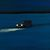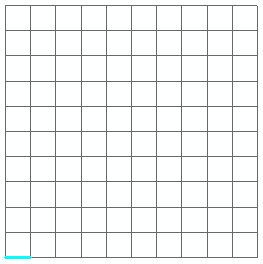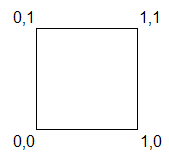# Convert grid lines to polygons

494
4
06-16-2022 06:49 PM
Labels (1)by
Frequent Contributor II

I have a SQL script that quickly generates grid line features, for testing purposes, using whatever grid dimensions I need: Generate SDO_GEOMETRY grid using SQL

• The result is a spatial view (can be easily exported as a feature class).
• I'm aware that there are GP tools that can generate grids too. But for my use case, I like this SQL option.
• The lines are split at the intersections. So this 10x10 grid has 220 lines:Question:

Is there a way to convert the grid lines to polygons? (i.e. a polygon for each grid cell)

I tried creating a polygon envelope FC and then I looked for a GP tool that could split the polygon via multiple lines. But I haven't found a GP tool that can do that yet.

Tags (1)
1 Solution

Accepted SolutionsNew Contributor III

The "feature to polygon" tool can do it no?

4 Repliesby
Frequent Contributor II

I suppose one way to do it would be to modify the existing query:

Details:

(1) Keep only the horizontal lines. Exclude the vertical lines.

`where    startpoint_y = endpoint_y`

Here's a sample horizontal line:

```  OBJECTID STARTPOINT_X STARTPOINT_Y ENDPOINT_X ENDPOINT_Y SHAPE
---------- ------------ ------------ ---------- ---------- --------------
219            0            0          0          1 [SDO_GEOMETRY]```

(2) Add an additional outer query where we generate 5 vertices (a polygon) from the existing 2 vertices (from the original horizontal line):

`startpoint_x, --vertex 1 (original vertex from the horizontal line)startpoint_y,endpoint_x,   --vertex 2 (original vertex from the horizontal line)endpoint_y,endpoint_x,   --vertex 3endpoint_y+1,startpoint_x, --vertex 4startpoint_y+1,startpoint_x, --vertex 5 (same as vertex 1) https://gis.stackexchange.com/q/433083/62572startpoint_y,`

(3) Just like with the lines query, construct a polygon geometry by concatenating the coordinates together into WKT.

`sdo_geometry('polygon(('||startpoint_x||' '||startpoint_y||', '||endpoint_x||' '||endpoint_y||', '||endpoint_x||' '||(endpoint_y+1)||', '||startpoint_x||' '||(startpoint_y+1)||', '||startpoint_x||' '||startpoint_y||'))',26917) as shape`

(4) Now, the coordinates look like this (the vertex direction is counterclockwise; vertex 1 and 5 are both 0,0):(5) This is what the full query looks like:```--create or replace view sdo_geom_grid_polygons_vw as (
select
objectid,
'polygon(('||startpoint_x||' '||startpoint_y||', '||endpoint_x||' '||endpoint_y||', '||endpoint_x||' '||(endpoint_y+1)||', '||startpoint_x||' '||(startpoint_y+1)||', '||startpoint_x||' '||startpoint_y||'))'        as wkt,
sdo_geometry('polygon(('||startpoint_x||' '||startpoint_y||', '||endpoint_x||' '||endpoint_y||', '||endpoint_x||' '||(endpoint_y+1)||', '||startpoint_x||' '||(startpoint_y+1)||', '||startpoint_x||' '||startpoint_y||'))',26917) as shape
from
(

with dimension as (
select 0 as point from dual
union all
select level
from dual
connect by level <= 10
), points as (
select
a.point as startpoint,
b.point as endpoint,
c.point as fixed
from dimension a
cross join dimension b
cross join dimension c
where b.point - a.point = 1
)
select
cast(rownum as number(38,0)) as objectid,
startpoint_x,
startpoint_y,
endpoint_x,
endpoint_y
from
(
select
startpoint as startpoint_x,
fixed as startpoint_y,
endpoint as endpoint_x,
fixed as endpoint_y
from points
union all
select
fixed as startpoint_x,
startpoint as startpoint_y,
fixed as endpoint_x,
endpoint as endpoint_y
from points
)
order by startpoint_x, startpoint_y, endpoint_x, endpoint_y
)
where
startpoint_y = endpoint_y --get horizontal lines only
--);```byMVP Esteemed Contributorby
Frequent Contributor II

Yeah, that's the source of my query. I mention it in the link in the original post.New Contributor III

The "feature to polygon" tool can do it no?